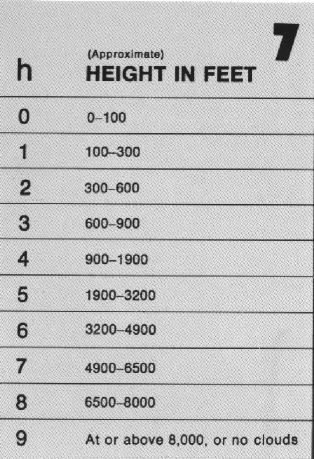## Current Air Temperature

The current air temperature plotted to the nearest whole degree Fahrenheit.

## Dew Point Temperature

The dew point temperature plotted to the nearest whole degree Fahrenheit.

## Sky Cover

The total amount of clouds in tenth. Plotted in the station circle according the the following table.## Wind Speed And Direction

The wind direction is plotted as the shaft of an arrow extending from the station circle toward the direction from which the wind is blowing. The wind speed is plotted as feathers and half-feathers representing 10 and 5 knots, on the shaft on the wind direction arrow. See the following table.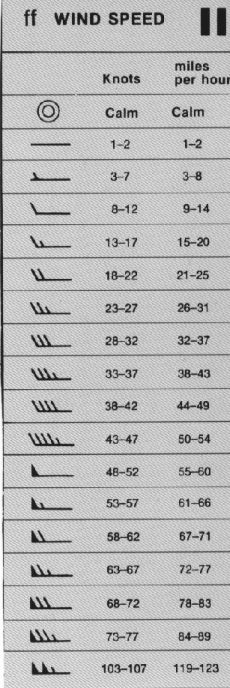## Visibility

The visibility plotted in fractions of a mile. For values above 10 miles, the values are omitted from the map.

## Sea Level Pressure

The barometric pressure in tenths of millibars reduced to sea level. The initial 9 or 10 and the decimal points are omitted.

ex: plotted = 982, actual = 998.2 mb
ex: plotted = 012, actual = 1001.2 mb

## Pressure Tendency

The pressure change in the past 3 hours preceding the observation. Plotted to the nearest tenth of a millibar. The plotted symbol characterizes the change in pressure according to the below chart.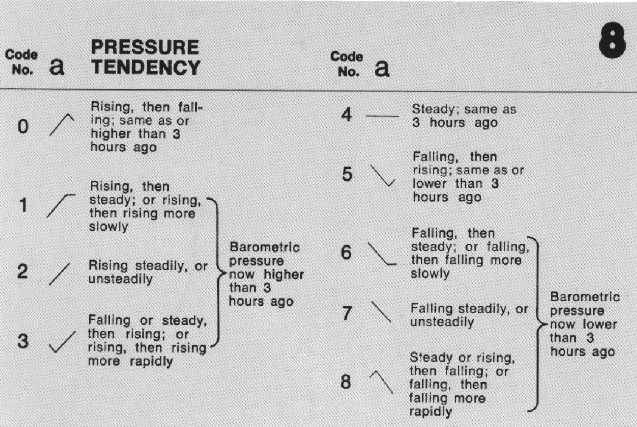## Past Weather

The most significant past weather since the last observation. The symbols are shown in the following table.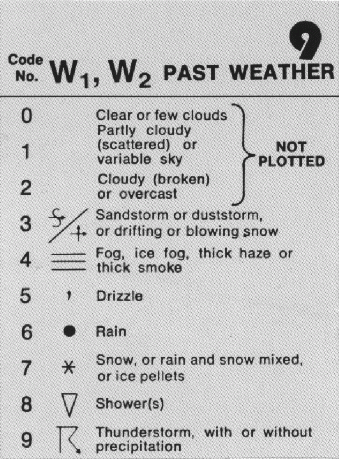## 6-Hour Precipitation Totals

The amount of precipitation during the past 6-hours in millimeters.

11 millimeters equals approximately 0.45 inches.

## Cloud Type

The predominate cloud type for the three levels (low clouds, middle clouds, high clouds). Each type are plotted with a symbol found in the following table.

#### Low Clouds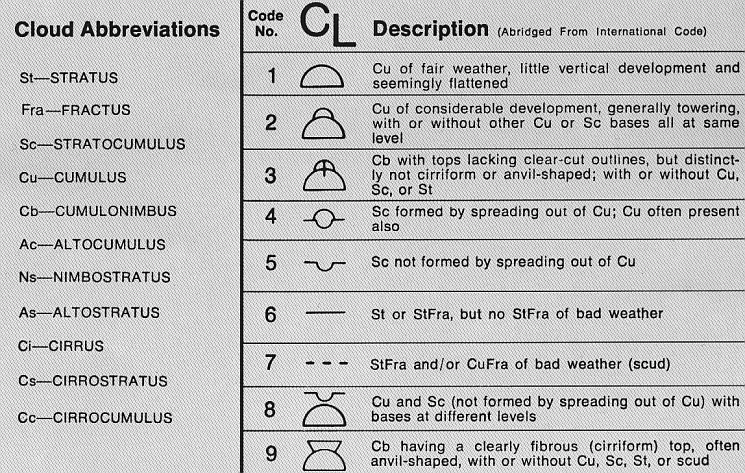#### Middle Clouds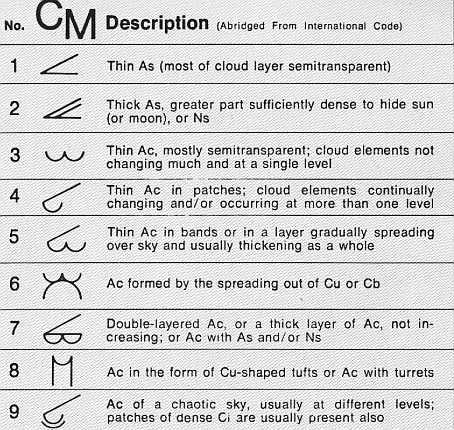#### High Clouds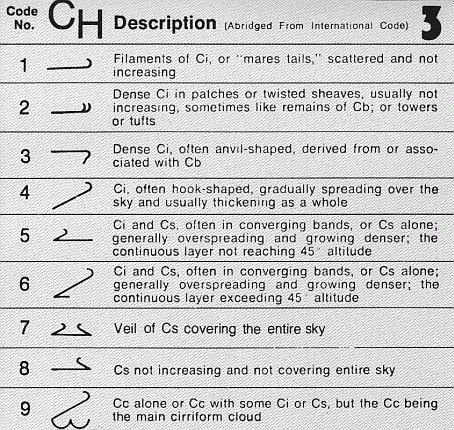## Fraction of Sky Cover

The fraction of the sky covered by low and middle clouds. Observed in tenth and plotted according to the code table below.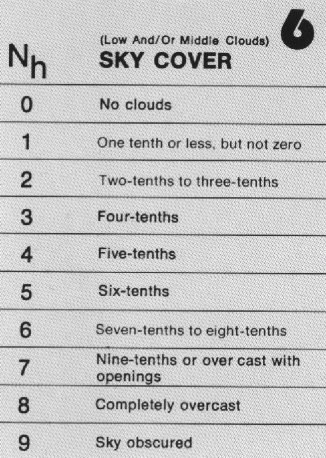## Height of Cloud Base

The height above the ground of the base of the lowest cloud. Observed in feet and plotted according to the code table below.